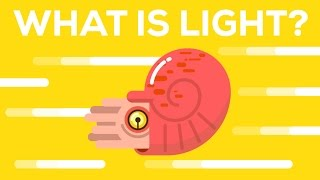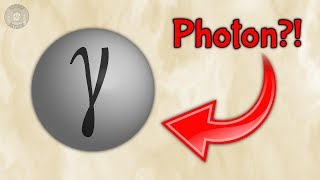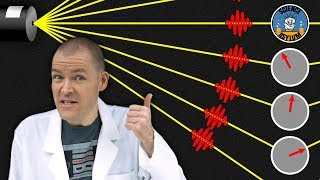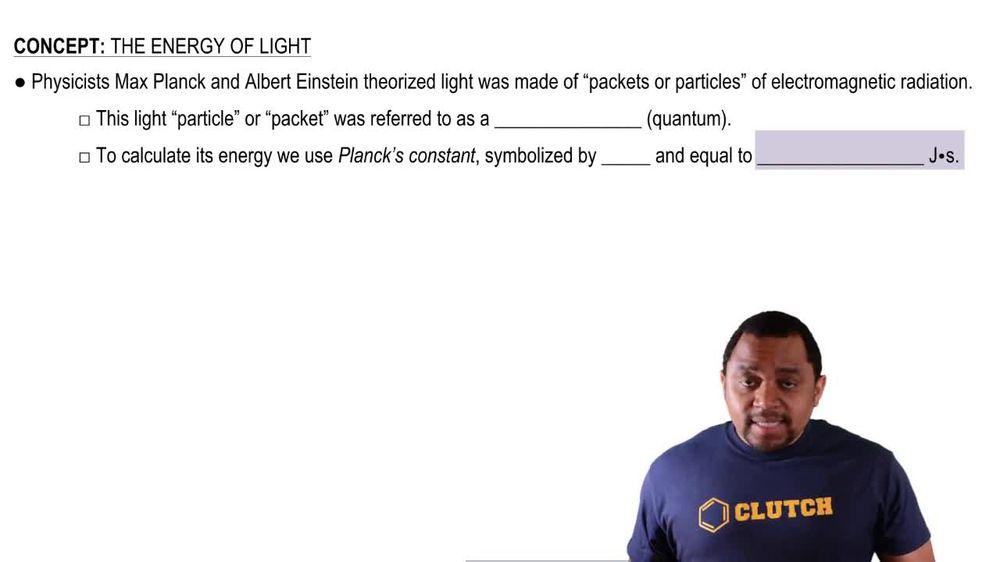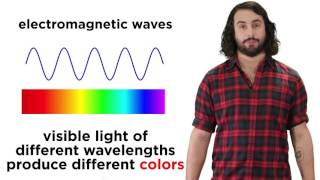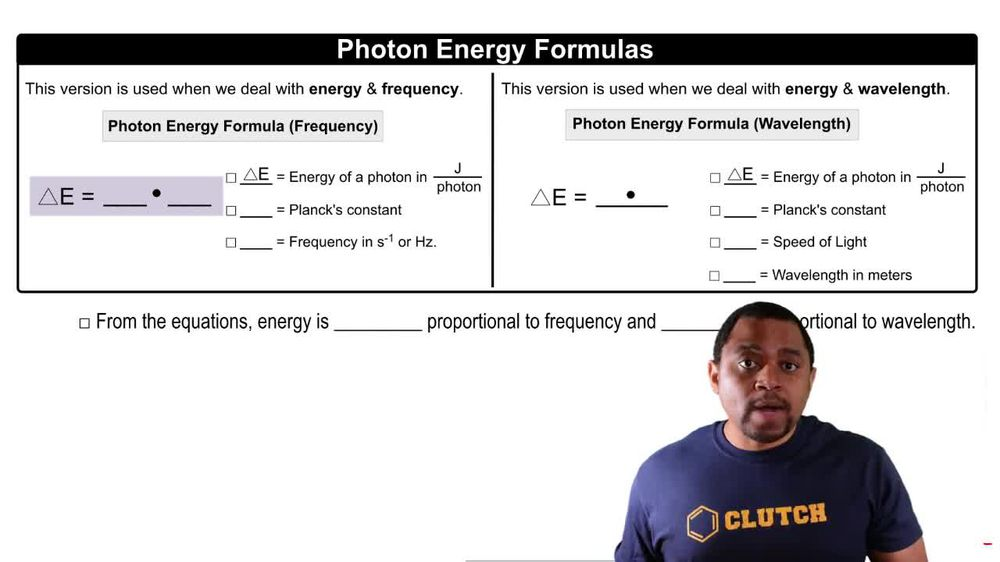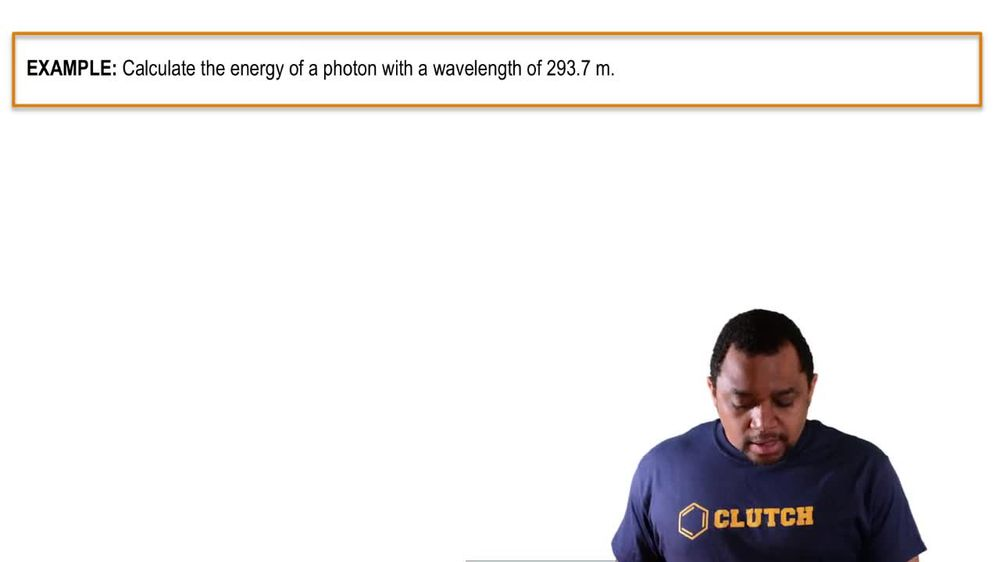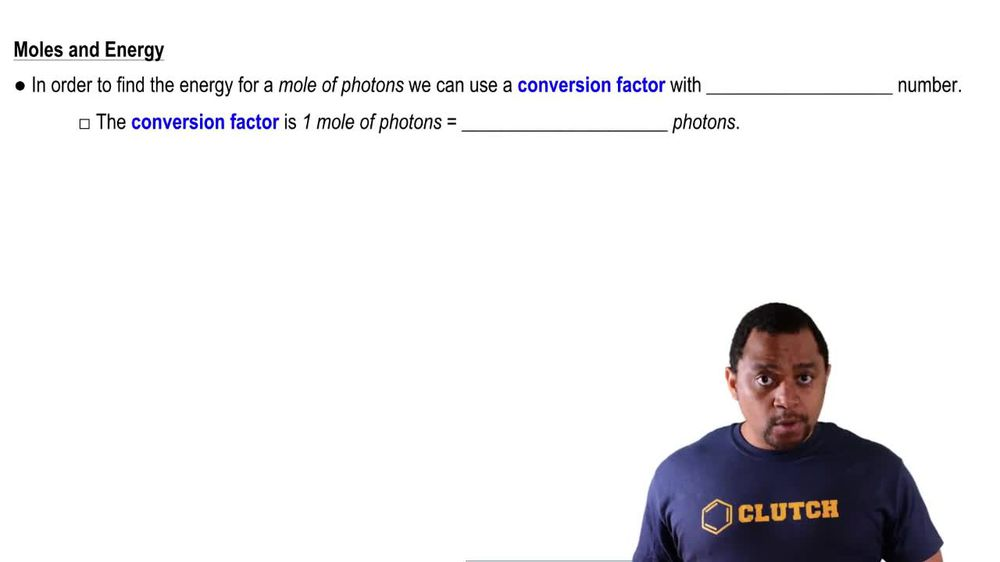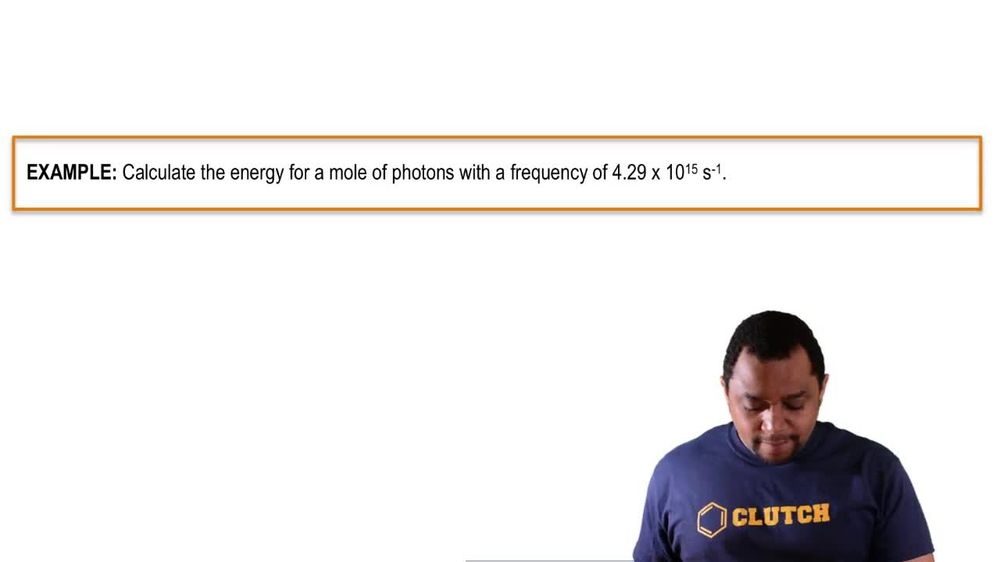Start typing, then use the up and down arrows to select an option from the list.
1. 9. Quantum Mechanics2. The Energy of Light# The Energy of Light

by Jules Bruno
218 views
1
1
So when it comes to calculating the energy of light in the form of ah photon, we have two different formulas that we can rely on and realize only one of them has a purple box around it, so that one is the more commonly used formula. And that's the one you should keep in mind and memorize. So we're gonna say here the first version is used when we deal with energy and frequency. So here is going to say that change in energy or energy of a photon in jewels per photon equals plank's constant, which is H times frequency, which is, in seconds, inverse or hurts. Remember, frequency uses the same on Greek symbol mu. Now the other one we use when we're dealing with calculating the energy of a photon when given energy and wavelength. In this one, we're gonna say changing energy or energy of a photon in jewels over photons equals planes constant still. But now it's times the speed of light, divided by wave length in meters. So just remember two different formulas, one we use when we're dealing with frequency and energy, and the other one we use when we're dealing with wavelength and energy. Now, from the equations realized that this tells us that energy is directly proportional to frequency, meaning that if your energy is going up, your frequency is going up and it would be inversely proportional inversely proportional toe wavelength. That means that if your energy or frequency are increasing, that means wavelength would happen. The would have to be decreasing. So just keep in mind the relationships that we have between now energy, frequency and wavelength.